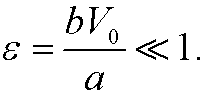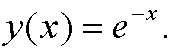# 3. Motion in a nonlinear resistive medium.

Suppose that a body with mass m and initial velocity V0 is moving rectilinearly in a medium that affects the body with the motion resistancewhere v=v(t), t>0, is the speed of the body at time t and a and b are positive constants withNewton’s second law then gives that the equation of motion for the body isRemark: If b=0 thenScaling: Introduce the dimensionless variablesThenand thus the differential equation is transformed towhereRemark 1: The unperturbed problemhas the solutionRemark 2: The perturbed problem has the solutionHint: make the substitution z=1/y.